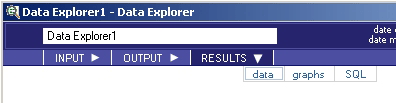# Data Explorer - RESULTS - Data - Teradata Warehouse Miner

## Teradata Warehouse Miner User Guide - Volume 1Introduction and Profiling

Product
Release Number
5.4.4
Published
July 2017
Language
English (United States)
Last Update
2018-05-03
dita:mapPath
wbc1492033894304.ditamap
dita:ditavalPath
ft:empty
dita:id
B035-2300
Product Category
Software
1. On the Data Explorer dialog box, click on RESULTS.
2. Click on data (note that the RESULTS tab will be grayed-out/disabled until after the analysis is completed).
Data Explorer > Results > DataResults data, if any, is displayed in a data grid as described in RESULTS Tab.

The Data Explorer analysis always creates a table for each descriptive statistical analysis specified. The structure of these output tables are specified below. Those columns in bold below comprise the Unique Primary Index (UPI).

Data Explorer Values Output Table
Name Type Definition
xdb VARCHAR(30) Database that contains the table that contains the column to be analyzed.
xtbl VARCHAR(30) Table that contains the column to be analyzed.
xcol VARCHAR(30) Column (variable) to be analyzed.
xtype VARCHAR(30) Data type of this variable.
xcnt FLOAT Total number of occurrences of this variable.
xnull FLOAT Total number of rows where this variable takes on a null value.
xunique FLOAT Total number of rows where this variable takes on a unique value.
xblank FLOAT Total number of rows where this variable is blank.
xzero FLOAT Total number of rows where this variable is equal to 0.
xpos FLOAT Total number of rows where this variable has a positive value.
xneg FLOAT Total number of rows where this variable has a negative value.
Data Explorer Statistics Output Table
Name Type Definition
xdb VARCHAR(30) Database that contains the table that contains the column to be analyzed.
xtbl VARCHAR(30) Table that contains the column to be analyzed.
xcol VARCHAR(30) Column (variable) to be analyzed.
xcnt FLOAT Total number of occurrences of this variable.
xmin FLOAT Minimum value of the variable. Created only if the Minimum Value option is selected.
xmax FLOAT Maximum value of the variable. Created only if the Maximum Value option is selected.
xmean FLOAT Arithmetic mean of the variable. Created only if the Mean Value option is selected.
xstd FLOAT Standard deviation of the variable. Created only if the Standard Deviation option is selected.
xskew FLOAT Skewness of the variable. Created only if the Skewness option is selected.
xkurt FLOAT Kurtosis of the variable. Created only if the Kurtosis option is selected.
xste FLOAT Standard error of the variable. Created only if the Standard Error option is selected.
xcv FLOAT Coefficient of variance of the variable. Created only if the Coefficient of Variance option is selected.
xvar FLOAT Variance of the variable. Created only if the Variance option is selected.
xsum FLOAT Sum of the variable. Created only if the Sum option is selected.
xuss FLOAT Uncorrected sums of squares of the variable. Created only if the Uncorrected Sums of squares option is selected.
xcss FLOAT Corrected sums of squares of the variable. Created only if the Corrected Sums of squares option is selected.
Data Explorer Frequency Output Table
Name Type Definition
xdb VARCHAR(30) Database that contains the table that contains the column to be analyzed.
xtbl VARCHAR(30) Table that contains the column to be analyzed.
xcol VARCHAR(30) Column (variable) to be analyzed.
xval VARCHAR(256) Distinct values of the frequency variable.
xcnt FLOAT Count of the number of rows that the frequency variable is equal to the distinct value specified in xval.
xpct FLOAT Percentage of the total records where the frequency variable is equal to the distinct value specified in xval.
Data Explorer Histogram Output Table
Name Type Definition
xdb VARCHAR(30) Database that contains the table that contains the column to be analyzed.
xtbl VARCHAR(30) Table that contains the column to be analyzed.
xcol VARCHAR(30) Column (variable) to be analyzed.
xbin INTEGER Integer representing the bin number.
xbeg FLOAT Value that represents the beginning boundary for the bin of the column specified.
xend FLOAT Value that represents the ending boundary for the bin of the column specified.
xcnt FLOAT Number of records in the bin.
xpct FLOAT Percentage of total records that this bin represents.